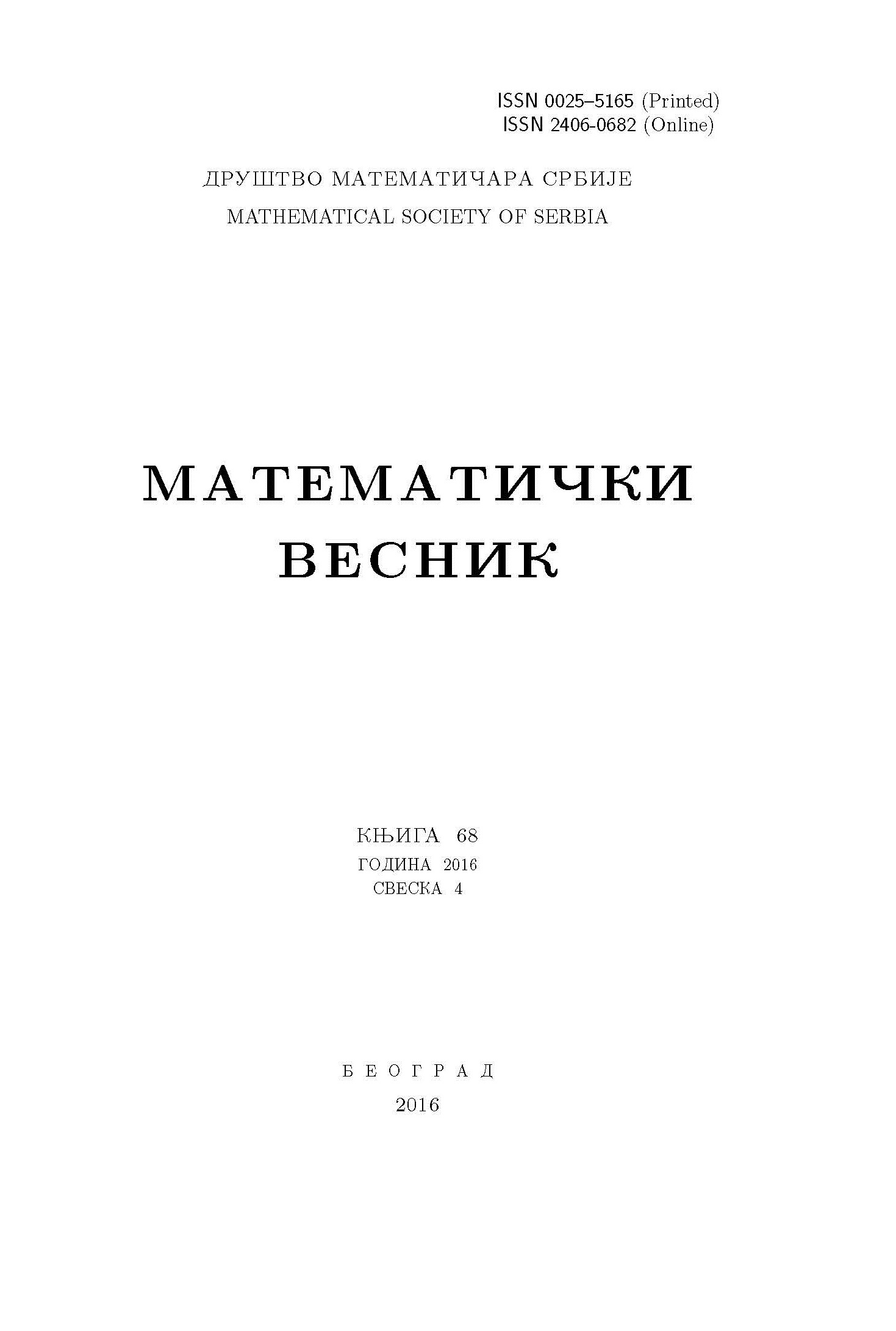﻿ Matematički Vesnik ﻿
 MATEMATIČKI VESNIK МАТЕМАТИЧКИ ВЕСНИКSOME PINCHING RESULTS FOR STATISTICAL SUBMANIFOLDS IN COSYMPLECTIC STATISTICAL MANIFOLDS M. Aquib, M. Aslam, M. N. Boyom AbstractIn this article, we discuss the curvature properties of statistical submanifolds in cosymplectic statistical manifolds with constant curvature. We also establish some pinching results for such submanifolds and hypersurfaces in cosymplectic statistical manifolds having constant curvature. As an application of the main result we also obtain an obstruction condition for such immersion.Keywords: Chen-Ricci inequality; dual connections; statistical manifolds; cosymplectic statistical manifolds. MSC: 53B05, 53B20, 53C40 Pages:  1$-$12

﻿